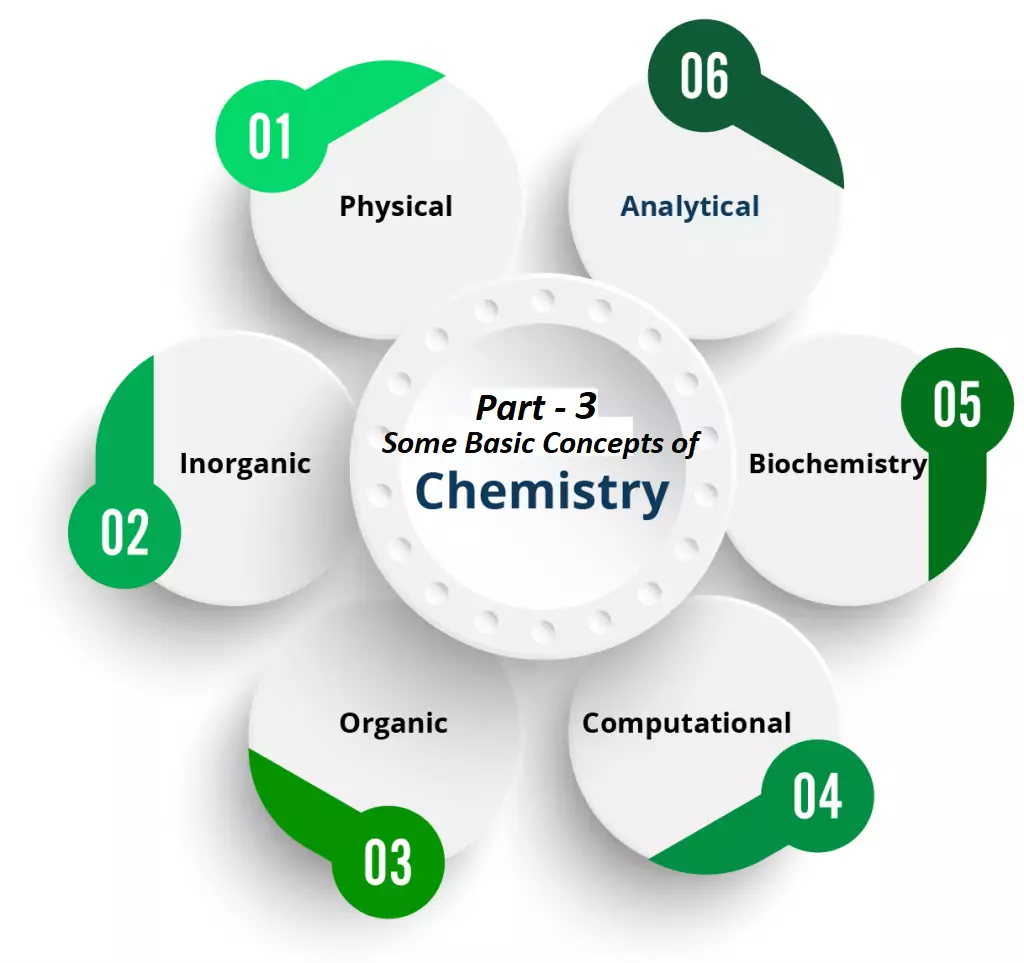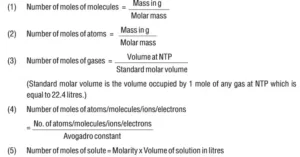# Some Basic Concepts of Chemistry Mole ConceptSome Basic Concepts of Chemistry Mole Concept

Atoms and Molecules: The atom is the smallest particle of an element. Molecules are the smallest particle of a substance. A molecule has all the properties of that substance.  Types of molecules Based on the type of atoms, there are two types of molecules – Homonuclear molecule and Heteronuclear molecule. A molecule containing only one type of atom is called a Homonuclear molecule. e.g. H2, O2, N2, O3 (ozone), etc Heteronuclear molecules contain different types of atoms. E.g. CO2, H2O, C6H12O6, NH3, etc. Based on the no. of atoms there are three types of molecules – monoatomic, diatomic, and polyatomic molecules.

• Monoatomic molecules contain only one atom. E.g. all metals, noble gases like He, Ne, Ar, etc.
• Diatomic molecules contain 2 atoms. E.g. H2, O2, N2, halogens (F2, Cl2, Br2, and I2).
• Polyatomic molecules contain more than two atoms. E.g. ozone (O3), Phosphorus (P4), Sulphur (S8), etc.

Atomic Mass: It is deﬁned as the average relative mass of an atom of an element as compared to the mass of an atom of carbon 12 taken as 12.  Atomic mass is represented by u (uniﬁed mass).

Atomic mass unit (amu): 1/12th the mass of a C12 atom is called the atomic mass unit (amu).  i.e. 1 amu = 1/12 × mass of a C12 atom
= 1.66 × 10-24 g
= 1.66 ×10-27 kg
Today, ‘amu’ has been replaced by ‘u’ which is known as unified mass.

Gram Atomic Mass: It may be defined as the mass of 1-mole atoms of an element. For eg. Mass of one oxygen atom = 16 amu = 16/NA gms
Mass of NA Oxygen atom = 16/NA×NA = 16 gms

Average atomic mass: All most all the elements have isotopes. So, we can calculate an average atomic mass of an element by considering the atomic mass of the isotopes and their relative abundance. For e.g. Chlorine has two isotopes 35Cl and 37Cl in the ratio 3:1. So the average atomic mass Cl = (3×35 + 1×37)/4 = 35.5

Molecular mass: It is the sum of the atomic mass of the elements present in the molecule. For example: Molecular mass of CH4 = (1 × 12) + (4 × 1) = 16 u
Molecular mass of H2SO4 is calculated as: 2 × 1 + 32 + 4 × 16 = 98 u.
The next topic is one of the most important topics of Some Basic Concepts of Chemistry Mole Concept.

Mole (n): It is the amount of a substance that contains as many particles or entities as the number of atoms in exactly 12 grams of pure C-12.
1 mole of any substance contains 6.022 x 1023 atoms. This number is known as Avogadro number or Avogadro constant (NA or N0).
1 mole of a substance = Molar mass of substance = Avogadro’s Number of chemical units = 22.4L volume at STP of a gaseous substance.
e.g., 1 mole of CH4 = 16g of CH4 = 6.022 × 1023 molecules of CH4 = 22.4L at STP.
n =   Given Mass     =   Volume(at STP) =  x Particles    =  MV
Molecular Mass         22.4L                       NA             1000
1 mol of hydrogen atoms = 6.022×1023 atoms
1 mol of water molecules = 6.022×1023 water molecules
1 mol of sodium chloride = 6.022 × 1023 formula units of sodium chloride

Molar volume: It is the volume of 1 mole of any substance. At standard temperature and pressure (STP), the molar volume of any gas = 22.4 L (or, 22400 mL). i.e. 22.4 L of any gas at STP contains 1 mole of the gas or 6.022 x 1023 molecules of the gas and its mass = molar mass. For e.g.
22.4 L of hydrogen gas = 1 mole of H2 = 6.022×1023 molecules of hydrogen = 2 g of H2
1 Mole of atoms = Gram atomic mass or molar mass = 6.022 x 1023 molecules = 22.4 L at NTP (00C, 1atm) = 22.7 L at STP (00C, 1 bar).

Rules in Brief:Dear readers kindly go through the various numerical problems related to the topic Some Basic Concepts of Chemistry Mole Concept.